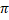# CBSE Solutions for Class 10 Maths

#### Select CBSE Solutions for class 10 Subject & Chapters Wise :

What is the diameter of the circle whose area is equal to the sum of the areas of two circles having radii 4 cm and 3 cm?

Answer :What is the area of a circle whose circumference is 8π?

Answer :What is the perimeter of a semicircular protractor whose diameter is 14 cm?

Answer :What is the radius of a circle whose perimeter and area are numerically equal?

Answer :Circumference of a circle is 39.6 cm. find its area.

Answer :

Circumference of circle = 2 πr = 39.6 cmCircumference of a circle is 22 cm. Find the area of its quadrant.

Answer :A circle whose area is equal to the sum of the areas of two circles of diameters 10 cm and 24 cm, Find the Diameter

Answer :If the area of a circle is numerically equal to twice its circumference, then find the diameter of the circle.

Answer :Find the perimeter of a square which circumscribes a circle of radius a cm.

Answer :Side of the square = 2  radius of circle = 2a cm

Then, Perimeter of the square = (4  2a) = 8a cm

What is the length of the arc of a circle of diameter 42 cm which subtends an angle of 60° at the centre?

Answer :All the vertices of a rhombus lie on a circle. Find the area of the rhombus, if the area of the circle is 1256 cm2. [Use π = 3.14] (2015OD)

Answer :

In rhombus, AB = BC = CD = AD
AC = BD = 2rIn Figure, ABCD is a square of side 14 cm. Semi-circles are drawn with each side of square as diameter. Find the area of the shaded region. [Use π = 22/7] (2016D)Answer :Area of shaded region = 2(Area of square) – 4(Area of semicircle) = 2 × 196 – 308 = 392 – 308 = 84 cm2

In Figure, arcs are drawn by taking vertices A, B and C of an equilateral triangle ABC of side 14 cm as centres to intersect the sides BC, CA and AB at BZ their respective mid-points D, E and F. Find the area of the shaded region. [Use π = 22/7  and  √3 = 1.73] (2011D)Answer :

ABC = BAC = ACB = 60°Match the radius with the resp. perimeters of circle

 1 12 A 36π 2 14 B 24π 3 16 C 28π 4 18 D 32π

Answer :

1-B, 2-C, 3-D, 4-A

Match the radius with the resp. perimeters of semi-circle

 1 2 A 6(π + 2) 2 4 B 2(π + 2) 3 6 C 8(π + 2) 4 8 D 4(π + 2)

Answer :

1-B, 2-D, 3-A, 4-C

Match the radius with the resp. perimeters of circle

 1 2 A 8π 2 4 B 12π 3 6 C 4π 4 8 D 16π

Answer :

1-C, 2-A, 3-B, 4-D

### Take a Test

Choose your Test :

### Chapter 12 : Areas Related to Circle

The 12th Chapter covers the concepts of perimeter (circumference) and area of a circle and apply this knowledge in finding the areas of two special ‘parts’ of a circular region known as sector and segment. And also it is about parts of circle, their measurements, and areas of plane figures.

### Browse & Download CBSE Books For Class 10 - All Subjects

The GSEB Books for class 10 are designed as per the syllabus followed Gujarat Secondary and Higher Secondary Education Board provides key detailed, and a through solutions to all the questions relating to the GSEB textbooks.

The purpose is to provide help to the students with their homework, preparing for the examinations and personal learning. These books are very helpful for the preparation of examination.

For more details about the GSEB books for Class 10, you can access the PDF which is as in the above given links for the same.